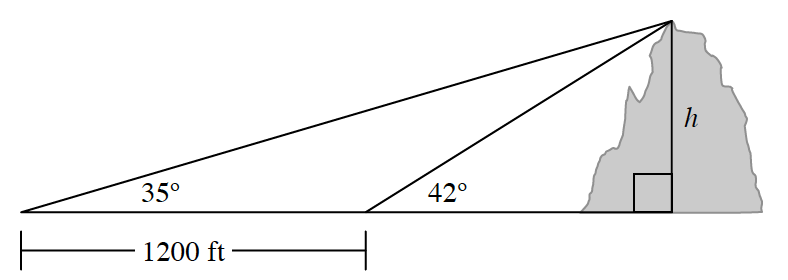### Home > PC3 > Chapter 10 > Lesson 10.1.3 > Problem10-64

10-64.

To determine the height, $h$, of Mount St. Melon in the Cantaloupe Mountains, two angle measurements were taken $1200$ feet apart along a direct line toward the mountain. Using these measurements, calculate the height of the mountain.Label the horizontal leg of the small right triangle $x$.
Then write two equations using tangent, $x$, and $h$.
Solve this system of equations for $h$.